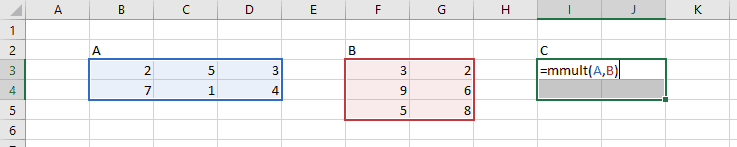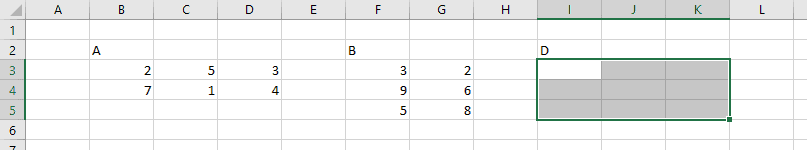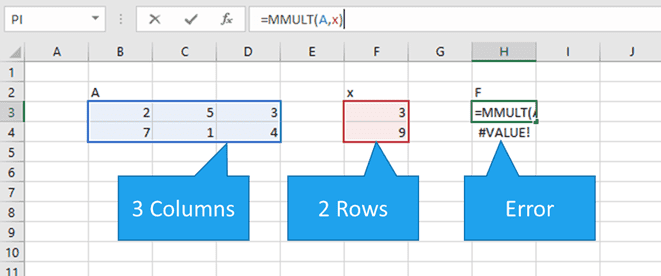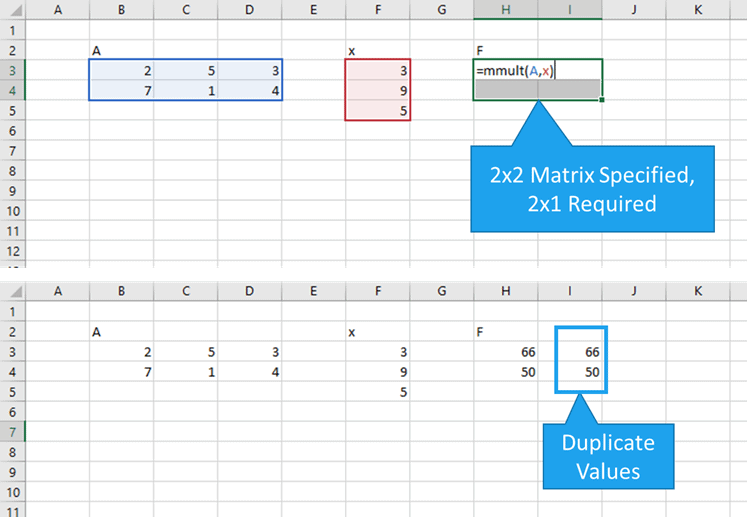# In Excel what is matrix multiplication

Since a worksheet is essentially a gigantic matrix, it’s no surprise that matrix multiplication in Excel is super easy.

## Matrix Multiplication in Excel with the MMULT function

You can multiply matrices in Excel thanks to the MMULT function. This array function returns the product of two matrices entered in a worksheet.

The syntax for the function is:

MMULT(array1,array2)

where array1 and array2 are the matrices to be multiplied.

## Matrix Multiplication Review

To perform matrix multiplication in Excel effectively, it’s helpful to remember how matrix multiplication works in the first place. So, let’s say we have two matrices, A and B, as shown below:The product of these two matrices (let’s call it C), is found by multiplying the entries in the first row of column A by the entries in the first column of B and summing them together. This is also known as the dot product. This single value becomes the entry in the first row, first column of matrix C.We continue doing this until we’ve found the dot product for each row and column combination.

Since we multiply the rows of matrix A by the columns of matrix B, the resulting matrix C will have a size of 2 x 2. Or more generally, the matrix product has the same number of rows as matrix A, and the same number of columns as matrix B.

Because of the way matrix multiplication works, it’s also important to remember that we can only multiply two matrices if the number of rows in B matches the number of columns in A.

Got it?

If not, that’s ok. Hopefully a few examples will clear things up. 🙂

## Excel Matrix Multiplication Examples

Let’s take the matrices from up above and find the product using matrix multiplication in Excel with the MMULT function:First, let’s find C, the product of AB.

Since MMULT is an array function, it will return values to more than one cell.

We know that the result is going to be a 2×2 matrix because the first matrix, A, has two rows and the second matrix, B, has two columns.

So we select an area on the worksheet 2 cells wide by 2 cells high:Next, with the area still selected, type the array formula and select the arguments (I assigned names to the matrices A and B):Finally, type CTRL+SHIFT+ENTER (because it’s an array formula) to return the resulting matrix product:What if, instead of calculating matrix C, by multiplying matrix A times B, we calculated a different matrix D, by multiplying matrix B times A?

Since the first matrix, B, has 3 rows, and the second matrix, A, has 3 columns, matrix D would have 3 rows and 3 columns. So, we would start with a selection 3 cells wide and high:Then, type in the formula for MMULT, selecting B as array1 and A as array2……to obtain matrix D:The MMULT function also works for multiplying a matrix (A) times an array (x). Of course, the rule still stands that the number of rows in x must match the number of columns in A.

The result is an array, F, that has 1 column and the same number of rows as A:## MMULT Errors in Excel

There are a few different ways that you can get errors in Excel with the MMULT function:

A #VALUE! error will occur when the number of columns in array 1 is not the same as the number of rows in array2.A #VALUE! Error will also occur if any of the elements in either array1 or array2 do not contain numbers.Although not always an error, MMULT can return some surprising results when the output area for the function is larger than required.

Adding extra columns causes the results to be duplicated:Adding extra rows to the MMULT array causes an #N/A! error to be displayed, although it’s only displayed in the extra cell:## Matrix Multiplication Limits in Excel

There are no software-imposed limits to the size of matrix that can be multiplied in current versions of Excel. Basically, you can multiply matrices as large as you want provided you have enough RAM in your computer.

However, if you’re still using Excel 2003 or earlier, you’ll be restricted to an output of 5046 cells when using the MMULT function (roughly a 71×71 matrix).

Even if you are in newer versions of Excel, there is one caveat: you cannot create an array that fills an entire column of a worksheet. Since there are 1,048,576 rows in a worksheet in Microsoft Excel 2007 and later, I guess this does constitute a limit on matrix multiplication in Excel.

But good grief! If you have that much data, you may want to consider some alternatives. 🙂

This article from Microsoft support has some great information on the limitations of Excel when working with arrays.